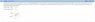# Moving point charges - help debugging

1MileCrash

See attachment

## The Attempt at a Solution

I was successful with finding a solution. But, only because I tried the practice version of that (where you can see the answers) and noticed the equation I derived gave angles that, when summed with the correct answer, yielded 180*.

Can someone tell me why?

START:

Since Bead 1 is stationary, we know its electric field will be in the direction of the positive x axis.

$E_{1} = \frac{1}{4\pi\epsilon_{0}} \frac{q_{1}}{r^{2}} \hat{i}$

While Bear 2 can be moved, thus its field will be a function of theta.

$E_{1} = \frac{1}{4\pi\epsilon_{0}} \frac{q_{2}}{r^{2}}cos(\theta) \hat{i} + \frac{1}{4\pi\epsilon_{0}} \frac{q_{2}}{r^{2}}sin(\theta) \hat{j}$

Thus, we add these two vector equations together for net E, and take the magnitude. After a bit of algebra we get:

$E^{2}(4\pi\epsilon_{0})^{2} = q^{2}_{1} + 2q_{1}q_{2}cos(\theta) + q^{2}_{2}cos^{2}(\theta) + q^{2}_{2}sin^{2}(\theta)$

By factoring out the q2 squared and noting the trigonometric identity,

$E^{2}(4\pi\epsilon_{0})^{2} = q^{2}_{1} + 2q_{1}q_{2}cos(\theta) + q^{2}_{2}$

$E^{2}(4\pi\epsilon_{0})^{2} - q^{2}_{1}- q^{2}_{2}= 2q_{1}q_{2}cos(\theta)$

$\frac{E^{2}(4\pi\epsilon_{0})^{2} - q^{2}_{1}- q^{2}_{2}}{2q_{1}q_{2}}= cos(\theta)$

For a final formula of:

$arccos(\frac{E^{2}(4\pi\epsilon_{0})^{2} - q^{2}_{1}- q^{2}_{2}}{2q_{1}q_{2}}) = \theta$

However, the value of the number within the arccos function is the negative of what it should be.

This gives me the answer correctly:

$arccos(- \frac{E^{2}(4\pi\epsilon_{0})^{2} - q^{2}_{1}- q^{2}_{2}}{2q_{1}q_{2}}) = \theta$

Why?

#### Attachments

•circlecharge.jpg
10.2 KB · Views: 353

Homework Helper
Gold Member
$E_{1} = \frac{1}{4\pi\epsilon_{0}} \frac{q_{2}}{r^{2}}cos(\theta) \hat{i} + \frac{1}{4\pi\epsilon_{0}} \frac{q_{2}}{r^{2}}sin(\theta) \hat{j}$

Consider the direction of the electric field of bead 2 and decide on the signs of its x and y components.

1MileCrash
I think I understand you.

While cos(45) is positive, at an angle 45 B's electric field is pointing down and towards the left.

Right?

Homework Helper
Gold Member
Yes1MileCrash
Thank you very much.